# Ball and Beam Part 2

Posted on at

today i write about Ball and Beam Project which was the topic of my last blog.Today i proceed it.

Physical setup and Lagrangian equation of system:

We declare some parameters and give some constant value and then use Lagrangian equation for mass of ball.

ball = m = 0.11 kg

radius of ball = r = 0.015 m

lever arm offset = d = 0.03 m,

gravitational acceleration = g = 9.8 m/s^2,

length of the beam = L = 1.0 m,

ball's moment of inertia = J = 9.99e-6 kg.m^2,

ball position coordinate=r,

(alpha)  beam angle coordinate,(theta)  servo gear angle

The Langrangian equation for this system is defined as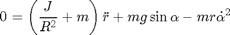linearize  this equation at alpha = 0.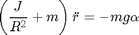equation 1

The equation which relates the beam angle to the angle of the gear can be approximated as linear by the equation below:

Sinα/ Sinθ=d/L

We consider Sinα=α and Sinθ=θ now putting this value in above equation

α/ θ=d/L

α= θd/L

now put this value in equation 1

we get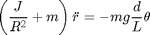Now take laplace transform

We get,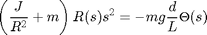Rearranging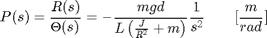State space model:

State space model of this system is defined as we consider two state variables ball position ‘r’ and velocity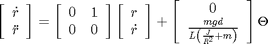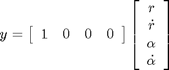Code

s = tf('s');P_ball = -m*g*d/L/(J/R^2+m)/s^2H = -m*g/(J/(R^2)+m);

A = [0 1 0 0

0 0 H 0

0 0 0 1

0 0 0 0];

B = [0 0 0 1]';

C = [1 0 0 0];

D = ;

ball_ss = ss(A,B,C,D)

System response using MATLAB Command:

In this section we will check the transfer function,system response at step input and then plot them to check stability.we see that our system is not stable so first we stable it then design PID controller in order to improve the response of our system as efficient as possible.

Stability:Response of this system was open loop so by giving unity gain feedback our system becomes stable.    Code     sys_cl=feedback(C*P_ball,1);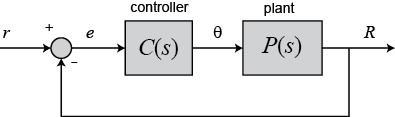Now for the third and the last part stay connected with me.